# <This tutorial was last updated in 2015. A new and better version (2018) is out, also including arbitrary heights for the target. click here>

In this tutorial, I’ll demonstrate how to implement an example of simple trajectory motion in Unity3D. In our scene, we will have a plane and a cube. The cube will jump to a target position that is randomly assigned on every jump command. The calculations will be based on an initial velocity and for simplicity’s sake, the projectile will land on the same height as its origin. The whole tutorial can be found on my public GitHub repository.

We will practically calculate the initial velocity required to launch the cube with a given angle to the target position. Let’s begin, shall we?

## 1 - Setting Up the Environment

• Create a new project. Open up the scene. Set camera position to `(0, 0, -15)` and rotation to `(30, 0, 0)`.
• Add a `Plane`, reset its transform and adjust its scale to `(2, 2, 2)`. Add a material to color the plane black.
• Add a `Cube`. Place it on position `(0, 0.5, 0)`. Add a `Rigidbody` component to it. Add a material to color it green.
• Create a C# script: “Launcher.cs”. Attach the script to the cube game object.
• Create an empty GameObject and name it to “Target”. Set its position to `(6, 0, -6)`
• I used a prefab from another game of mine for the target bullseye. You can simply use a sprite with a giant red X or circle on it to visualize the target position in the scene.

Here’s how the scene should look like now.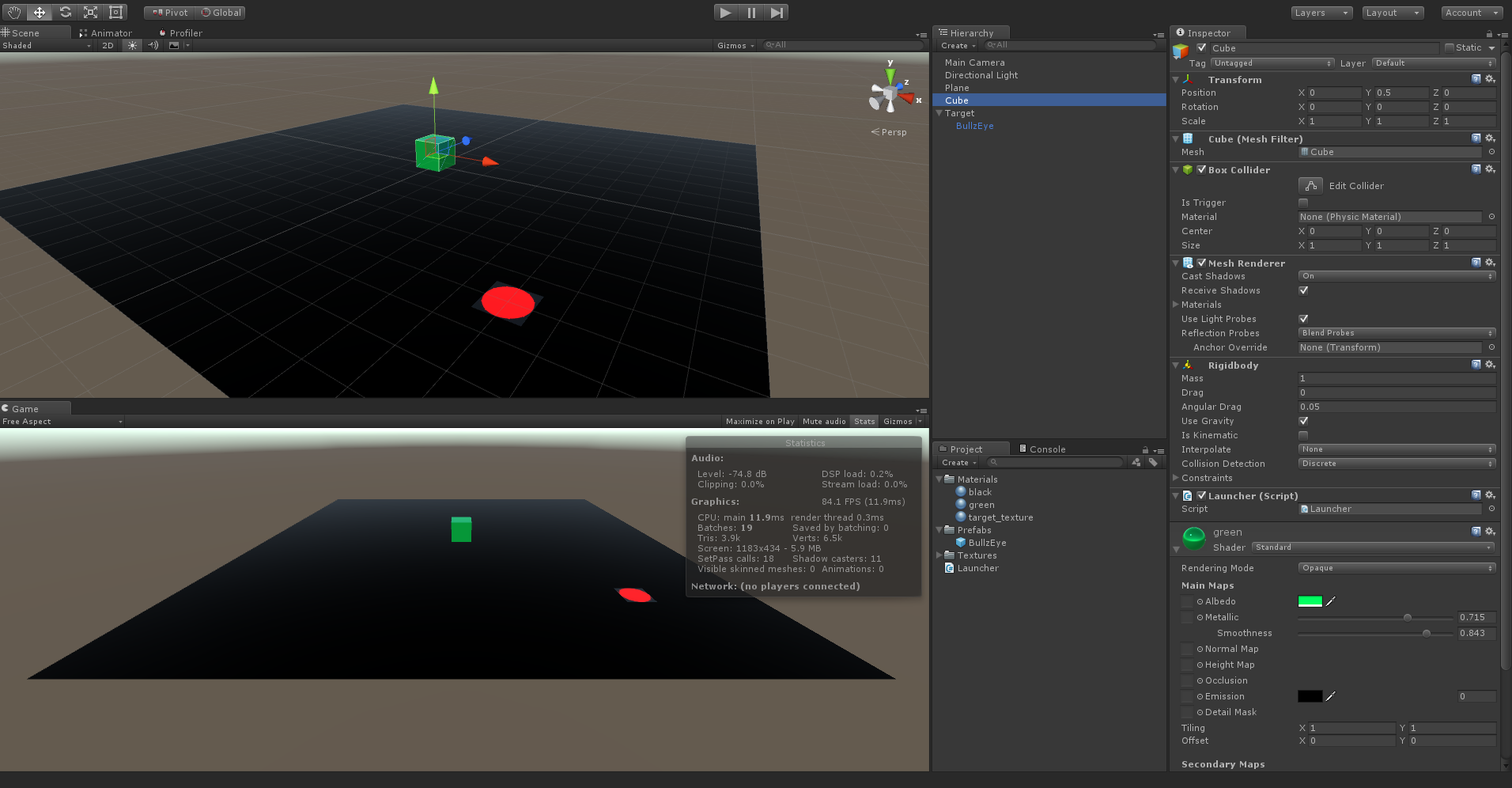Scene Setup

## 2 - Setting Up Target Locations

### Variables

• We are going to use a `bool _targetReady` switch for alternating between acquiring a new target, and shooting the cube to the target position when Spacebar is pressed.
• We will use a handle to the Transform GameObject (Bullseye prefab), named `_bullseye`. We will change its position when new target is acquired.
• We will use `Range float` variables. named `_targetRange` and `_angle`. The latter will be used for launching angle.

On the next step, we will implement acquiring a random position from a target pool, and then place the Bullseye prefab on the target. Here’s the code without the function implementations.

### AcquireTarget() Function

• We are gonna randomly choose points from a square’s vertices, middle points of edges and from the center point.
• After the point is designated, it will be scaled according to `_targetRange` variable.
• Finally, the bullseye’s position will be adjustted.

## 3 - Launching the Cube

When launching an object to a target position with a given shooting angle, it is quite handy to use local space vectors when defining the initial velocity vector. Unity has a great function for transforming a local direction into a global direction – `TransformDirection()`. Our approach will be quite simple:

• Turn the object to face the target position.
• Calculate required initial velocity using some high school physics.
• Calculate Vz and Vy – forward and up components of the initial velocity – and create the local velocity vector.
• Transfrom local space velocity vector into global space.
• Apply the initial velocity – Launch the object!

### Turning the Object and the Local Space

First, we will make the cube face the newly acquired target position.

When the coordinate system is changed from Global to Local from the editor (second of the Toggles near the basic toolbar) you can see the blue Z axis is coming out of the cube and turned to the target position when we call the `LookAt()` function. You can toggle between local and global space to see the different axis orientations.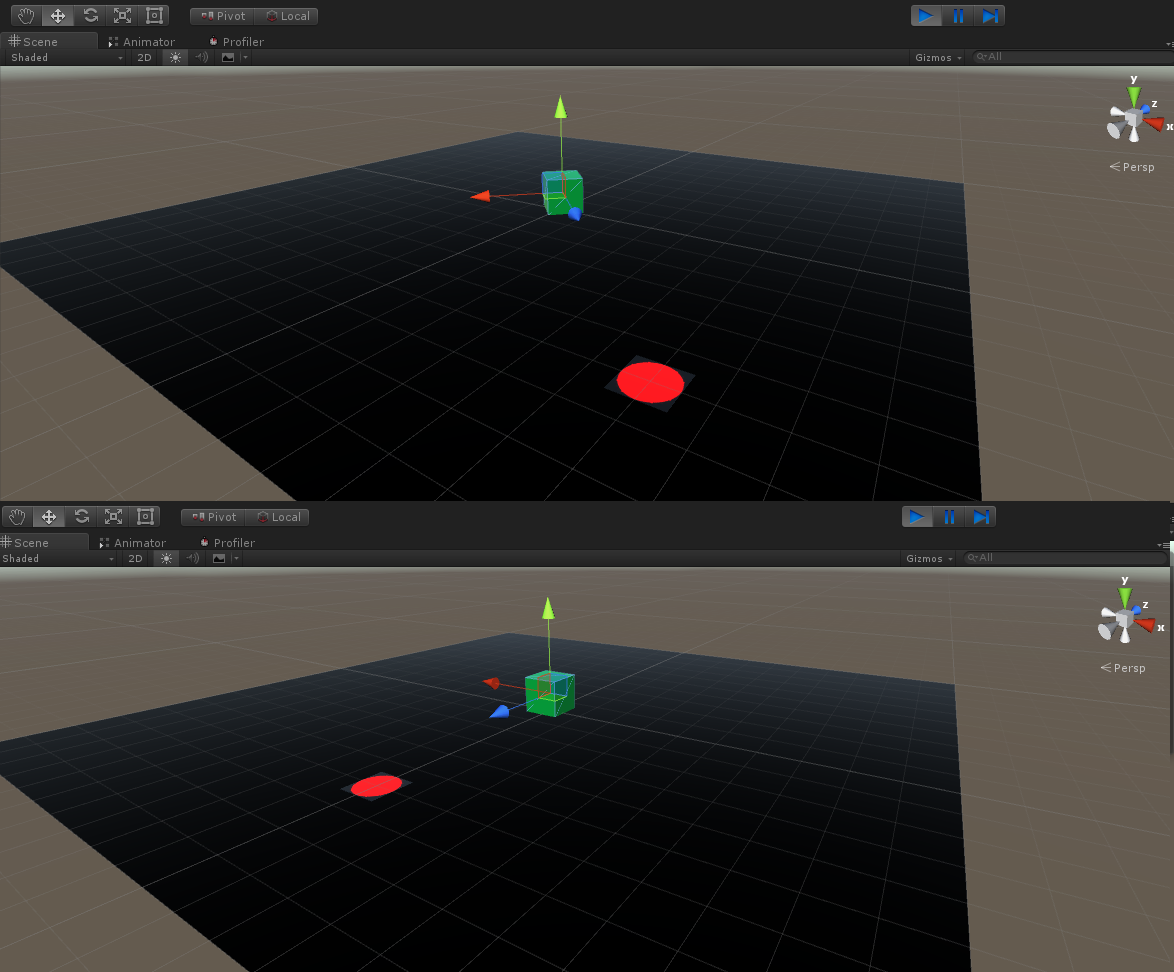Cube facing the target as seen in Local Space

Turning the cube to the target using `LookAt()` function will come quite handy when calculating the required initial velocity for launching as it will reduce the problem to a 2D space, so we can accomplish the trajectory motion by only calculating the local Z and Y components of the intial velocity. Before we do that, let’s have a look at the physics!

### Physics calculations

Below is a depiction of a trajectory motion.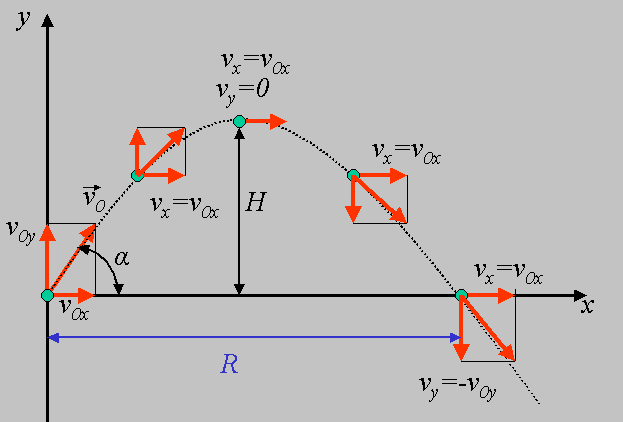Analyzing the initial velocity we get the following equations:

• (1) V0y = V0 * Sin(α)
• (2) V0x = V0 * Cos(α)

Let’s say this motion occurs over T time and the gravitational accelration is G. As the horizontal velocity V0x doesn’t change over time, we can see that

• (3) R = V0x * T

At the time T/2, the object will be at its peak point. The horizontal distance H will be traveled by the object during the remaining T/2 time, with V0y = 0 and with an acceleration of G. We can get use the free fall equation and get

• (4) H = 0.5 * G * T2

We also know that during the half time, the Y component of the initial velocity V0y reached zero from its initial value. Applying the Velocity = Acceleration x Time formula, we get

• (5) V0y - G * (0.5*T) = 0

Now that we have our base formulas, we can start playing with the formulas to get a useful final formula.

If we leave T in (5), we get

• (6) T = 2V0y / G

and plug T in (3)

• (7) R = 2V0xV0y / G

Finally plugging (1) and (2) into the equation (7), we get

• (8) R = 2V02Cos(α)Sin(α) / G

Using the “trigonometric angle transformation formula: half angles” and leaving V0 alone on equation (8), we finally get

• (9) V0 = √RG / Sin(2α)

We can now calculate the initial velocity, since we know all the unknowns:

• R = `Vector3.distance(pos, target);`
• G = `-Phsyics.gravity.y;`
• α = `float _angle;`

Lets translate all this into C# code. Since the `Mathf.Sin()` and `Mathf.Cos()` functions take angles as radians, we will need some conversions – i.e. will use `Mathf.Deg2Rad` for that.

There we go, our cube jumps to the target!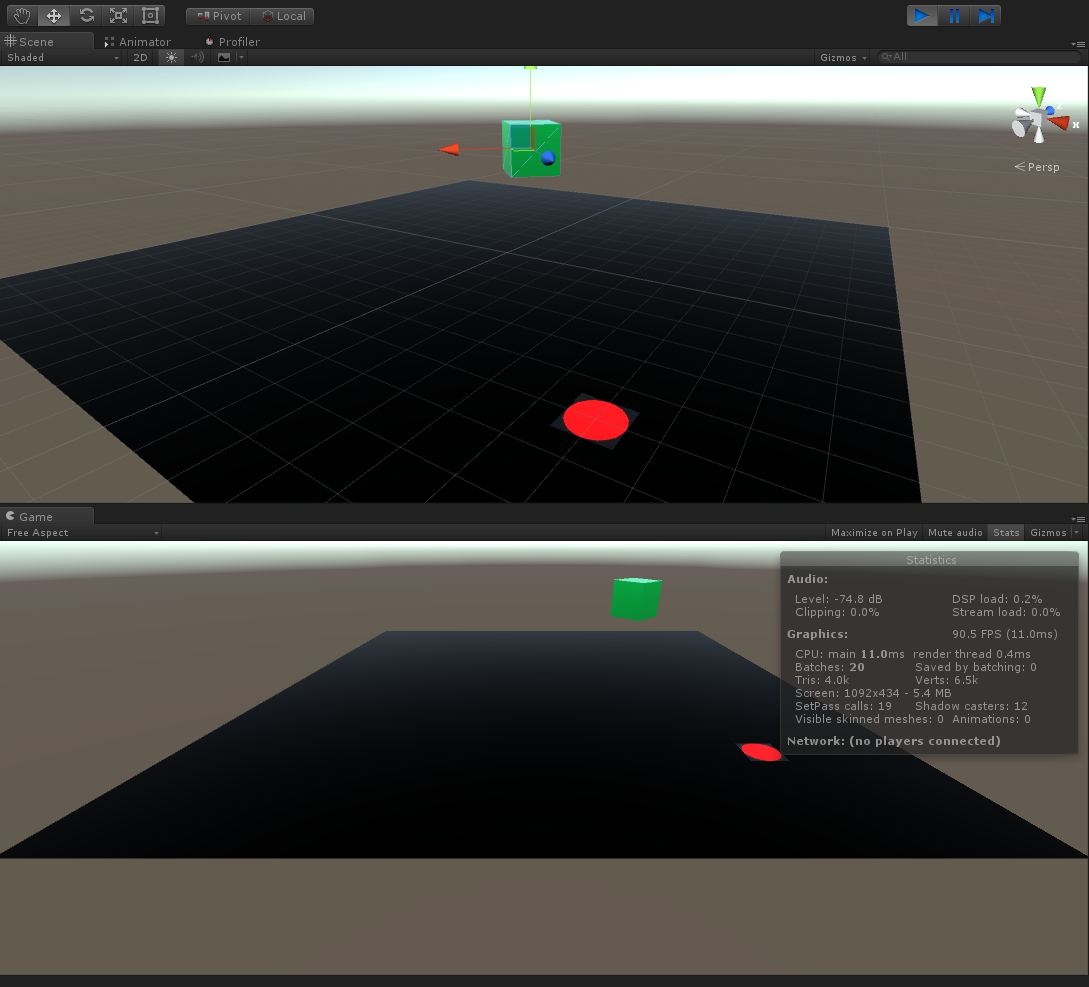## 4 - Bonus: Trajectory Rotation

If you are shooting objects like arrows or missiles, you’ll need to rotate them mid air to make it look like a real arrow or missile trajectory. To demonstrate what I mean, I’ll use a capsule to launch, instead of a cube.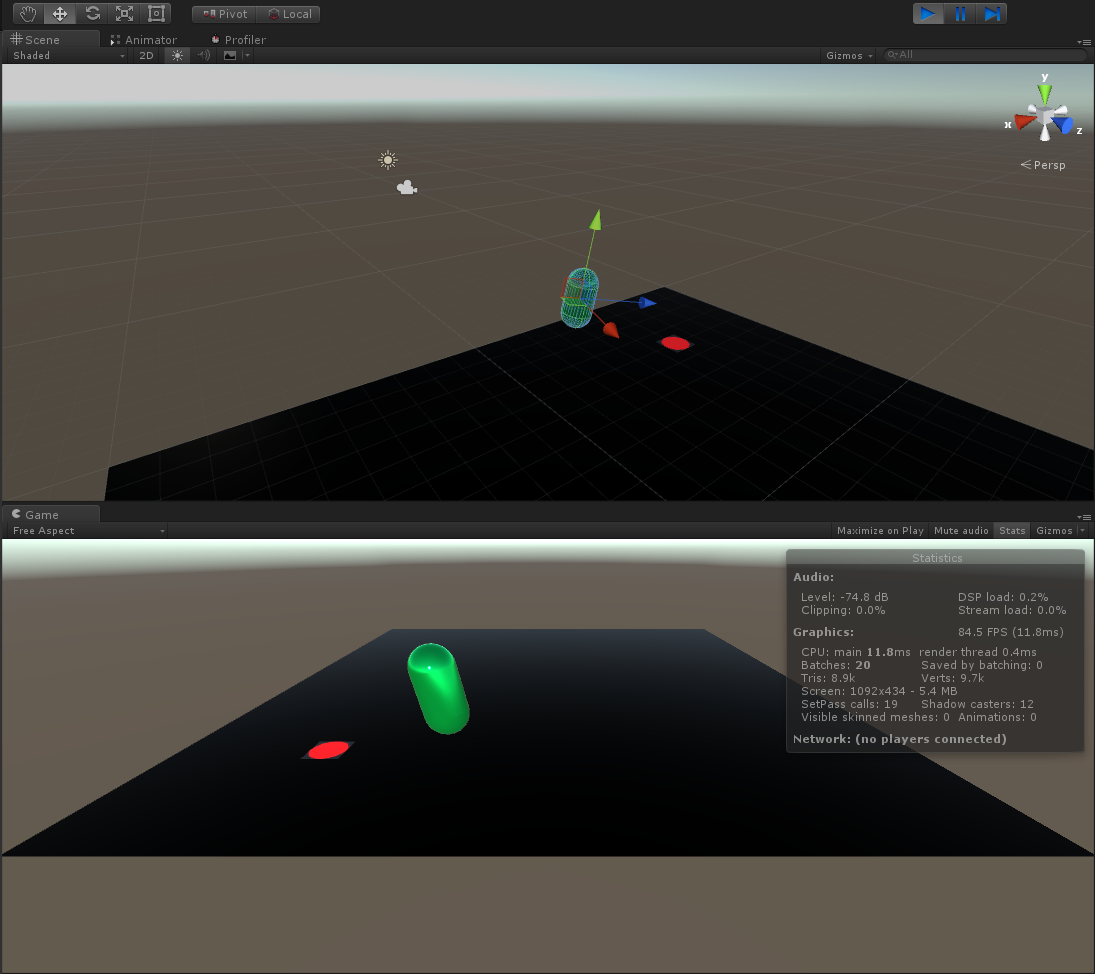As seen here, the capsule is launched with the rotation returned by the `LookAt()` function and it keeps its rotation during the trajectory motion. This is the “NO” scenario below.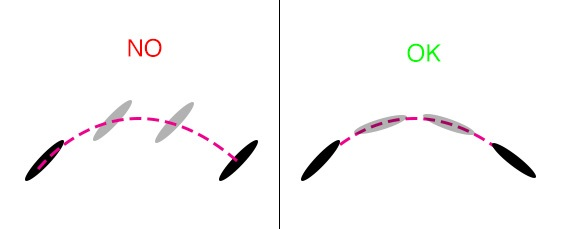To rotate the capsule to give it a more realistic look, we will create a new script named “Rotation.cs” and rotate the projectile during the course of trajectory motion.

There is one more arrangement to be made. To use the capsule like a missile or an arrow, we need an initial X rotation of 90 degrees, i.e. the projectile must be parallel to the ground as its initial orientation. Otherwise, the capsule will act similar to the missile in this scenario. To mitigate that,

• Create an empty game object.
• Add the capsule as a child to the empty object.
• Remove `rigidbody`, `capsule collider` and script components from the capsule.
• Rotate capsule on the X axis by 90 degrees.
• Add `rigidbody` and `capsule collider` and the Launcher.cs and Rotation.cs scripts to the empty game object.
• Set axis of the `capsule collider` as `Z-Axis`.
• Don’t forget to set the Launcher script’s editor variables!

There we go, a more realistic launch!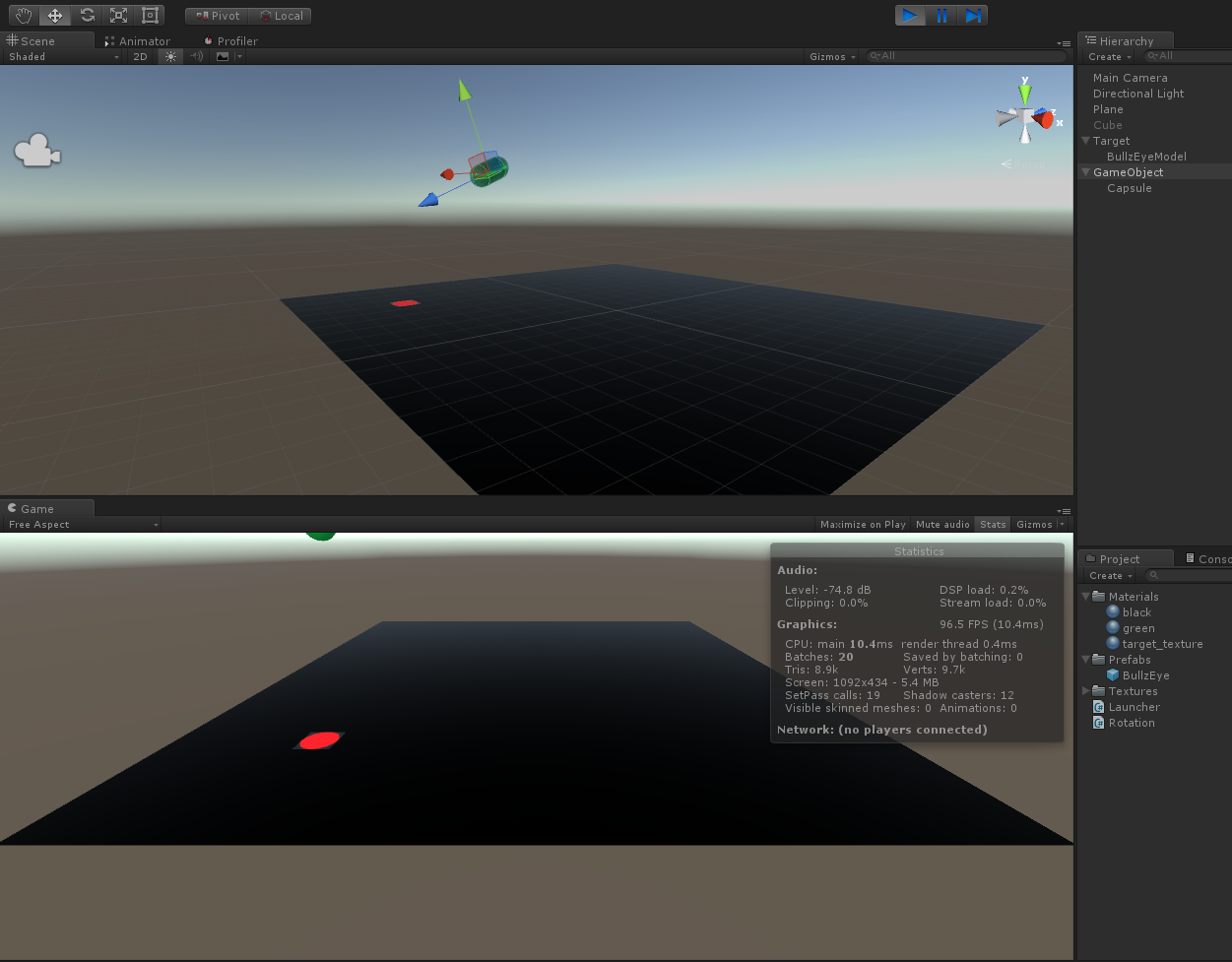See it in action on WebGL here.